# Chapter 13:PHASE EQUILIBRIUM:VAPOUR-LIQUID EQUILIBRIUM OF MIXTURES¶

## Example 13.1, Page No:605¶

In :
import matplotlib.pyplot as plt
import numpy as np

#Variable declaration
# Take freon 22 as component 1 and Freon 12 as component 2
# (a). y-x diagram at 40 oC
P1sat=15.335; # Saturation pressure of Freon 22 at 40oC in bar
P2sat=9.607; # Saturation pressure of Freon 12 at 40oC in bar
from pylab import *
figure(1); # For Plotting y-x Diagram
%matplotlib inline

#Calculation for (a)
a=P1sat/P2sat;
x1=linspace(0,1.0,3);
y1=(a*x1)/(1+x1*(a-1)); # y Function
plt.plot (x1,y1,'-*b',label='y1');
plt.plot (x1,x1,'-<r',label='x1'); # plot comment
plt.title ("(a).y-x diagram for the mixture at 40 oC");
plt.xlabel(" x1 ");
plt.ylabel(" y1 ");
plt.legend(loc='upper left')

#Result for (a)
print "(a). y-x diagram at 40 oC","\n figure 1"
plt.show();

#Calculation for (b)
# (b). p-x-y diagram at 40 oC
# By using the following relation calculate p value for various value of x1,y1
# p=(x1*P1sat)+(1-x1)*P2sat
x1=[0,0.2,0.5,0.8,1];
y1=[0,0.285,0.615,0.865,1];
p=[9.607,10.7526,12.471,14.1894,15.335];
figure(2);
plt.plot (x1,p,'-*b',label='Liquid out')
plt.plot(y1,p,'-<r',label='Vapour');
plt.title ("(b).P-y-x diagram for the mixture at 40 oC");
plt.xlabel(" x1 & y1 ");
plt.ylabel(" p in bar ");
plt.legend(loc='upper left')

#Result for (b)
print "(b). p-x-y diagram at 40 oC","\n figure 2"
plt.show();

#Calculation for (c)
# (c).t-x-y diagram at 10 bar
# for any value of x1 at p=10 bar, the bubble temperature can be found by trial and error from the following relation
# p=10 bar =(x1*P1sat)+(1-x1)*P2sat
T1sat=23.7; # Saturation temperature of Freon 22 at 10 bar in  oC
T2sat=41.6; # Saturation temperature of Freon 12 at 10 bar in  oC
# Thus, for x1=0.5, we find that t=31 oC.
x1=0.5; # Let assume
P1sat=12.186; # Saturation pressure of Freon 22 at 31oC in bar
P2sat=7.654; # Saturation pressure of Freon 12 at 31oC in bar
a=P1sat/P2sat;
y1=(a*x1)/(1+x1*(a-1)); # y Function
# For different value of x1 the values of t,y1 are calculated by above expression and given below
x1=[0,0.5,1];
y1=[0,0.614,1];
t=[41.6,31,23.7];
figure(3);
plt.plot (x1,t,'-*b',label='f');
plt.plot (y1,t,'-<r',label='g');
plt.title ("(c).t-y-x diagram for the mixture at 10 bar");
plt.xlabel(" x1 & y1 ");
plt.ylabel(" t in oC ");
plt.legend(loc='upper right');

#Result for (c)
print "(c).t-x-y diagram at 10 bar","\n figure 3"
plt.show();

(a). y-x diagram at 40 oC
figure 1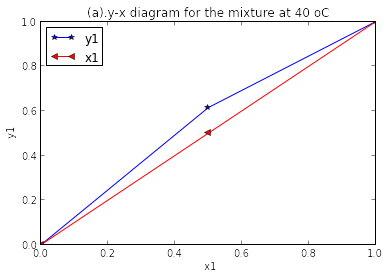(b). p-x-y diagram at 40 oC
figure 2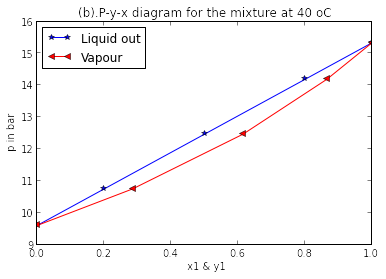(c).t-x-y diagram at 10 bar
figure 3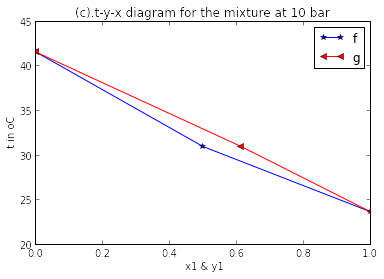## Example 13.2, Page No:612¶

In :
import math

#Variable declaration
T=573.15; # Temperature of the water with another liquid in kelvin
R=8.3144/18; # Characteristic gas constant
# (a).4 MPa
P_1=10; #  By Method II, The lowest possible pressure at which date available in steam table for 300 oC temperature in kPa
h_i=3076.5; # Specific enthalphy at P_1 in kJ/kg
s_i=9.2813; # Specific entropy at P_1 in kJ/kg K
# from superheat table at p=4 MPa and t=300 oC
hi=2960.7; # Specific enthalphy in kJ/kg
si=6.3615; # Specific entropy in kJ/kg K

#Calculation for (a)
fi=P_1*math.exp ((((hi-h_i)/T)-(si-s_i))/R); # Standard state fugacity of water

#Result for (a)
print "(a).4 MPa","\nStandard state fugacity of water = ",round(fi,2),"kPa   (round off error)"

#Variable declaration for (b)
# (b).equal to saturation pressure at 300 oC
Psat=8.581; # Saturation pressure at 300 oC in MPa
# From steam table at Psat=8.581 MPa and t=300 oC
hi=2749; # Specific enthalphy in kJ/kg
si=5.7045; # Specific entropy in kJ/kg K

#Calculation for (b)
fi=P_1*math.exp ((((hi-h_i)/T)-(si-s_i))/R); # Standard state fugacity of water
pisat=fi/(Psat*10**3); # fugacity coefficient

#Result for (b)
print "\n(b).Equal to saturation pressure at 300 oC","\nStandard state fugacity of water = ",round(fi,0),"kPa"
print "fugacity coefficient =",round(pisat,4)

#Calculation for (c)
# (c).10 MPa
# Applying Method I
viL=0.001404; # Specific volume at 300 oC in m^3/kg
fi=pisat*Psat*10**3*math.exp ((viL*(P_1-Psat)*10**3)/(R*T)); # Standard state fugacity of water
print "\n(c).10 MPa","\nStandard state fugacity of water = ",round(fi,0),"kPa"

(a).4 MPa
Standard state fugacity of water =  3591.45 kPa   (round off error)

(b).Equal to saturation pressure at 300 oC
Standard state fugacity of water =  6694.0 kPa
fugacity coefficient = 0.7801

(c).10 MPa
Standard state fugacity of water =  6745.0 kPa


## Exapmple 13.3, Page No:615¶

In :
import matplotlib.pyplot as plt
import numpy as np
import math
%matplotlib inline
import math
from __future__ import division

#Variable declaration
# Let take NH3 as component 1 and H2O as component 2
# (a) & (b)
# Calculation of f1sat = pi1sat*p1sat for ammonia
P_1=50; # low reference state pressure in kPa
P1sat=614.95; # Saturation Pressure of ammonia at 10 oC in kPa
h1sat=1453.3; # Specific enthalpy at 10 oC in kJ/kg
s1sat=5.2104; # Specific entropy at 10 oC in kJ/kg K
R=8.3144/17; # Characteristic gas constant
T=283; # Temperature in kelvin
# At 10 oC and P_1=50 kPa for ammonia
h_1sat=1499.2; # Specific enthalpy in kJ/kg
s_1sat=6.5625; # Specific entropy in kJ/kg K

#Calculation for (a)&(b)
f1sat=P_1*math.exp ((((h1sat-h_1sat)/T)-(s1sat-s_1sat))/R); # Standard state fugacity of Ammonia
# Calculation of f2sat = pi2sat*p2sat for water
P2sat=1.2276; # Saturation Pressure at 10 oC in kPa for water
pi2sat=1; # At low pressure for water
f2sat = pi2sat*P2sat; # Standard state fugacity of water
# Calulations of ViL/RT
# For ammonia and water at 10 oC
v1L=0.001601; v2L=0.001; # Specific volume in m^3/kg
v1L_RT=v1L/(R*T); v2L_RT=v2L/(R*T);
# Calculations of activity coefficients
# Expression for activity coefficients of ammonia and water become in given by respectively
# r_1=(y1*p/(x1*569.6))*exp (-4.34*10^-6*(p-p1sat)); for ammonia
#  r_2=(y2*p/(x2*1.2276))*exp (-7.65*10^-6*(p-p2sat)); for water
# The values thus calculated for r_1,r_2,lny_1,lnr_2 are calculated and plotted in window 1
# Note that the values of pyonting factors are negligibly small
x1=[0,0.2,0.3,0.4,0.5,0.6,0.8,1.0];
y1=[0,0.963,0.986,0.9958,0.9985,0.9993,0.9999,1.0];
lnr_1=[-3.1,-1.845,-1.295,-0.75,-0.33,-0.065,-0.035,-0];
lnr_2=[0,-0.1397,-0.2767,-0.507,-0.709,-0.952,-1.613,-2.2];
# similarly the excess function gE/RT and gE/x1x2RT are also calculated using the following expression respectively
# gE_RT=x1*lnr_1+x2*lnr_2; # the excess function from 12.51
# gE_x1x2RT=(lnr_1/x2)+(lnr_2/x1);
# since gE=0 & x1x2=0 both at x1=0 and x1=1. However its values in between x1=0 & x1=1
# By substituting these values in the above expression and given below
gE_RT=[0,-0.481,-0.582,-0.604,-0.5195,-0.4198,-0.2925,0];
gE_x1x2RT=[-3.1,-2.92,-2.83,-2.74,-2.65,-2.56,-2.38,-2.2];
from pylab import *
figure(1); # For Plotting  Diagram
plt.plot (x1,lnr_1,"-*b",label='ln r1');
plt.plot (x1,lnr_2,"-*g",label='ln r2');
plt.plot (x1,gE_RT,"r",label='gE/RT');
plt.plot (x1,gE_x1x2RT,"-*k",label='gE/x1x2 RT');
plt.title ("(a)&(b).Activity coefficients for NH3/H2O at 10 oC");
plt.xlabel(" x1  ");
plt.ylabel(" ln r ");
plt.legend(loc='lower right')

#Result for (a)&(b)
print "(a) & (b)","\nStandard state fugacity of Ammonia = ",f1sat,"kPa"
print "Standard state fugacity of water = ",f2sat,"kPa"
print "v1L/RT = ",v1L_RT,"(answer mentioned in the textbook is wrong)","\nv2L/RT = ",v2L_RT
print "\n(a)&(b).Activity coefficients for NH3/H2O at 10 oC"
plt.show();
print " figure 1 "
# As x1→0,x2→1,gE_x1x2RT→A=ln r_1^∞
# As x1→1,x2→0,gE_x1x2RT→B=ln r_2^∞
A=-3.1; B=-2.2; # THe Margules constants
print "The Margules constants ","A = ",A,"B = ",B
print "From figure 1 for ammonia/water mixture which is characteristic of systems with negative deviation from Roault law."
print "Because γi<=1   and   ln γi <=0"

#Calculations for (c)
# (c).
# Assuming ideal vapour phase, and at low pressures we have
# y1P=γ1*x1*p1sat; y2p=γ2* x2* p2sat;
# Now the activity coefficients can be found from Margules equations and given below
x1=[0,0.2,0.3,0.4,0.5,0.6,1.0];
y1=[0,0.963,0.986,0.9958,0.9985,0.9999,1.0];
p=[1.2276,8.6597,30.6598,54.6845,150.6458,278.1549,614.95];
# The ideal solution pressure
# PRaoult=x1*P1sat+x2*P2sat;
PRaoult=[1.2276,614.95];
x_1=[0,1]; # For Ideal solution pressure
from pylab import *
figure(2); # For Plotting  Diagram
plt.plot (x1,p,"-*r",label='p-x1');
plt.plot (y1,p,"-*b",label='p-y1');
plt.plot (x_1,PRaoult,"g",label='PRaoult');
plt.title ("(c).p-x-y diagram of NH3/H2O at 10 oC");
plt.xlabel(" x1  & y1 ");
plt.ylabel(" p, kPa   ");
plt.legend(loc='upper left')

#Result for (c)
print "\n\n(c).p-x-y diagram"
print "figure 2"
plt.show();
print "From figure 2 The actual pressure p < pRaoult. It is thus seen that the mixture has negative deviation  from Raoults law."

(a) & (b)
Standard state fugacity of Ammonia =  569.617676377 kPa
Standard state fugacity of water =  1.2276 kPa
v1L/RT =  1.15670577403e-05 (answer mentioned in the textbook is wrong)
v2L/RT =  7.22489552801e-06

(a)&(b).Activity coefficients for NH3/H2O at 10 oC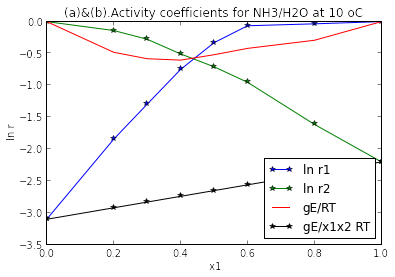figure 1
The Margules constants  A =  -3.1 B =  -2.2
From figure 1 for ammonia/water mixture which is characteristic of systems with negative deviation from Roault law.
Because γi<=1   and   ln γi <=0

(c).p-x-y diagram
figure 2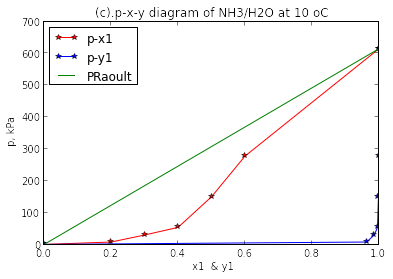From figure 2 The actual pressure p < pRaoult. It is thus seen that the mixture has negative deviation  from Raoults law.


## Example 13.4, Page No:624¶

In :
import math
from __future__ import division

#Variable declaration
x1=0.9; # mole fraction of NH3
x2=0.1; # Mole fraction of H2O
p=490.3; # Pressure in kPa
T=280.1; # Temperature in kelvin
lam12_11=-2131; lam21_22=-2726; # In kJ/kmol
R_1=8.3144; # Universal gas constant  in kJ/kmol K
# (a).Enthalpy of saturated liquid Mixture at L/B at bubble temperature
V1L=0.0016; V2L=0.001; #from properties of NH3 and H2O in m^3/kg

#Calculation for (a)
a=((V2L*18)/(V1L*17)) * exp (-lam12_11/(R_1*T));
b=((V1L*17)/(V2L*18)) * exp (-lam21_22/(R_1*T));
d_a=a*(lam12_11/(R_1*T**2)); d_b=b*(lam21_22/(R_1*T**2));
d_lnr1=(-(a*x2**2*d_a/(x1+(a*x2))**2))-(x2*d_b/(b*x1+x2))+(b*x1*x2*d_b/(b*x1+x2)**2);
d_lnr2=(-b*x1**2*d_b/(b*x1+x2)**2)-(x1*d_a/(x1+a*x2))+(a*x1*x2*d_a/(x1+a*x2)**2);x1=0.728; # By substituting these valuses in equation
h_E=-R_1*T**2*(x1*d_lnr1+x2*d_lnr2); # Heat of mixing
x1=0.9;
M=x1*17+x2*18; # Molecular weight
hE=h_E/M;
h1L=32.5; h2L=29.4; # in kJ/kg
hL=(x1*h1L)+(x2*h2L)+hE;# Specific enthalpy of the liquid mixture

#Result for (a)
print "(a).Enthalpy of saturated liquid Mixture at L/B at bubble temperature",
print "Specific enthalpy of the liquid mixture = ",round(hL,1),"kJ/kg"

#Variable declaration (b)
# (b).Enthalpy of saturated vapour at V in Equilibrium with liquid at L/B
# From property table of ammonia and water at 0 oC
T1=273.15; # Temperature in kelvin
p1sat=429.4; p2sat=0.6108; # Pressure in kPa
hfg1=1262.4; hfg2=2501.4;# specific enthalpy in kJ/kg
vg1=0.2895; vg2=206.3; # specific volume in m^3/kg
# Referring to fig 13.15 , we have
hb1=1262.4; hb2=2501.4;# specific enthalpy in kJ/kg
M=17;
# The crictical properties
Tc1=405.3; Tc2=647.3;# Temperature in kelvin
pc1=11.28; pc2=22.09; # Pressure in MPa

#Calculation for (b)
z1=(p1sat*vg1/(R_1*T1/M)); z2=(p2sat*vg2/(R_1*T/M));
A2_1=(0.4278/(pc1*10**3))*(Tc1/T1)**2.5; # Constants
B_1=(0.0867/(pc1*10**3))*(Tc1/T1); # Constants
h1R=R_1*(T1/M)*(((-3/2)*(A2_1/B_1)*log (1+(B_1*p1sat/z1)))+z1-1);
A2_2=(0.4278/(pc2*10**3))*(Tc2/T1)**2.5; # Constants
B_2=(0.0867/(pc2*10**3))*(Tc2/T1); # Constants
h2R=-0.2;
hc1=hb1-h1R; hc2=hb2-h2R; # Enthalpies at 0 oC
Cpo1=14.86; Cpo2=12.92; # In kJ/kg
A2_1=(0.4278/(pc1*10**3))*(Tc1/T)**2.5; # Constants
B_1=(0.0867/(pc1*10**3))*(Tc1/T); # Constants
A2_2=(0.4278/(pc2*10**3))*(Tc2/T)**2.5; # Constants
B_2=(0.0867/(pc2*10**3))*(Tc2/T); # Constants
y1=0.9999; y2=0.0001;
Tc=y1*Tc1+y2*Tc2;
z=0.957;
hR=R_1*(T/M)*(((-3/2)*(A2_1/B_1)*log (1+(B_1*p/z)))+z-1);
hV=y1*(hc1+Cpo1)+y2*(hc2+Cpo2)+hR;

#Result for (b)
print "\n(b).Enthalpy of saturated vapour at V in Equilibrium with liquid at L/B = ",round(hV,1),"kJ/kg   (roundoff error)"

#Variable declaration for (c)
# (c).Enthalpy of saturated vapour at D after complete vaporization of liquid at B/L
T=359.15; # In K
Cpo1=192.2; Cpo2=160.9; # In kJ/kg

#Calculation for (c)
A2_1=(0.4278/(pc1*10**3))*(Tc1/T)**2.5; # Constants
B_1=(0.0867/(pc1*10**3))*(Tc1/T); # Constants
A2_2=(0.4278/(pc2*10**3))*(Tc2/T)**2.5; # Constants
B_2=(0.0867/(pc2*10**3))*(Tc2/T); # Constants
y1=0.9; y2=0.1;
Tc=y1*Tc1+y2*Tc2;
z=0.9768;
hR=R_1*(T/M)*(((-3/2)*(A2_1/B_1)*log (1+(B_1*p/z)))+z-1);
hD=y1*(hc1+Cpo1)+y2*(hc2+Cpo2)+hR;

#Result for (c)
print "\n(c).Enthalpy of saturated vapour at D after complete vaporization of liquid at B/L = ",round(hD,1),"kJ/kg"

#Calculation for (d)
# (d).Latent Heat of Vapourization of this Liquid Mixture
hB=-0.2;
hD_hB=hD-hB; #Latent Heat of Vapourization of this Liquid Mixture

#Result for (d)
print "\n(d). Latent Heat of Vapourization of this Liquid Mixture = ",round(hD_hB,1),"kJ/kg mixture"

(a).Enthalpy of saturated liquid Mixture at L/B at bubble temperature Specific enthalpy of the liquid mixture =  -0.2 kJ/kg

(b).Enthalpy of saturated vapour at V in Equilibrium with liquid at L/B =  1280.1 kJ/kg   (roundoff error)

(c).Enthalpy of saturated vapour at D after complete vaporization of liquid at B/L =  1581.3 kJ/kg

(d). Latent Heat of Vapourization of this Liquid Mixture =  1581.5 kJ/kg mixture